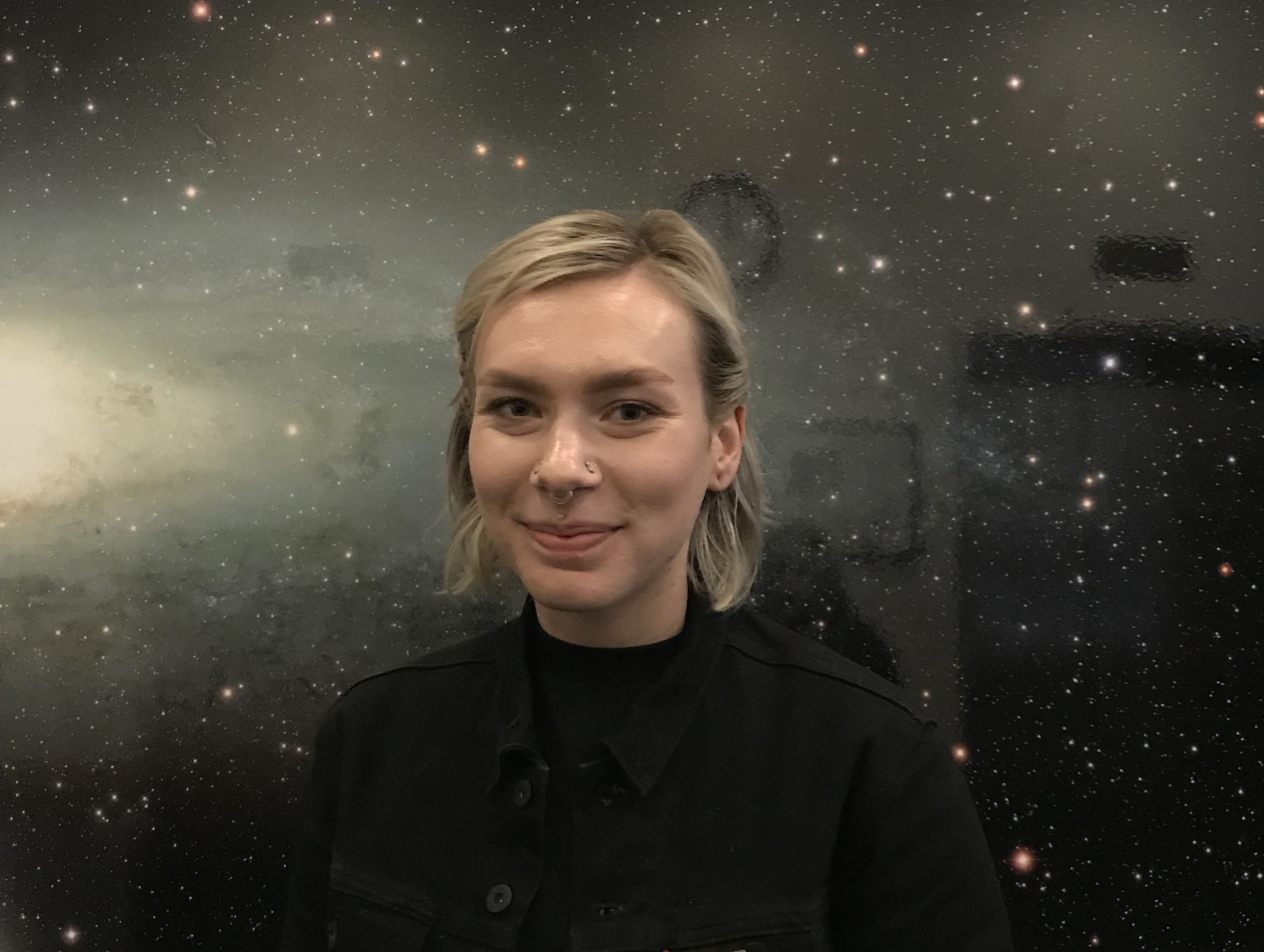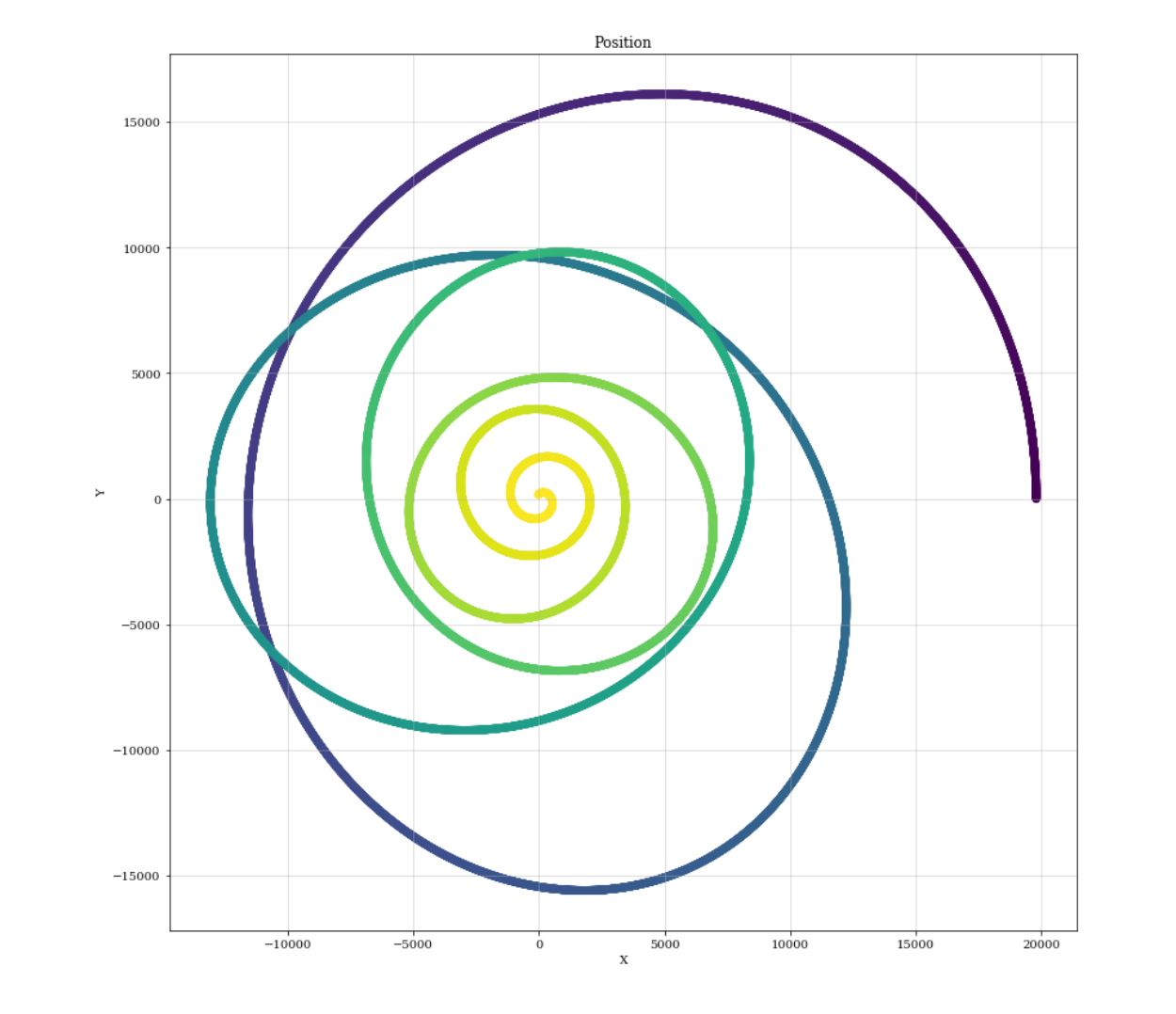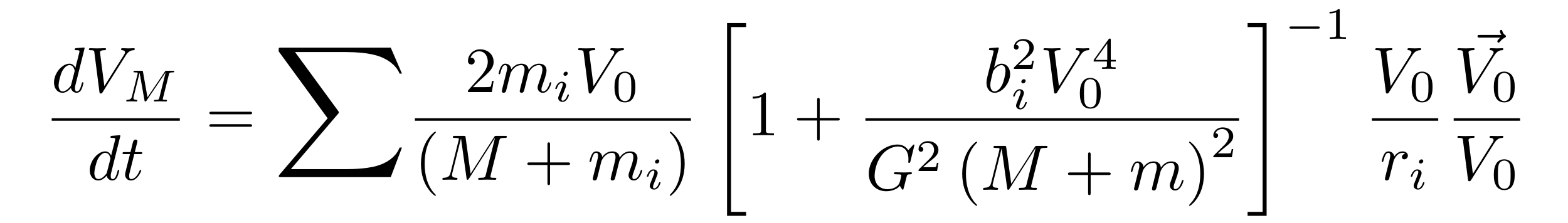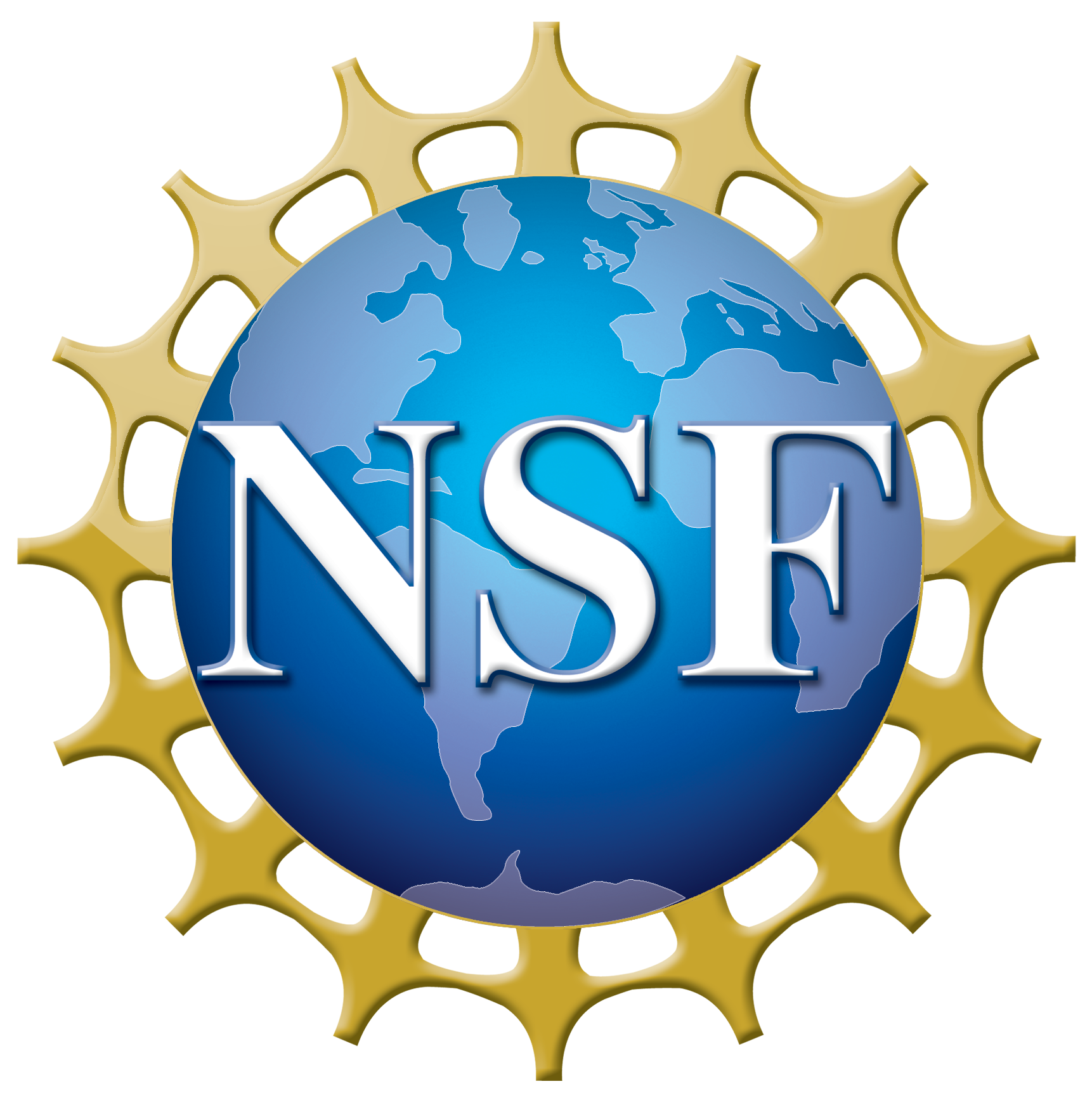## CIERA REU (Mentors: Dr. Luke Zoltan Kelley, Claude-Andre Faucher-Gigere) GalForm Group

 About Me Dynamical Friction Our Formula Our Galaxy Model Comparing Results Next StepsSophia is an Astronomy and Physics double major completing her Bachelor of Science degree at the University of Washington in Seattle. Sophia is in entering her Junior Year. She is interested in large structure, galaxy evolution, cosmology, and theory. Her main interests lie in the interdisiplinary aspects of astrophysics, combining mathematics, numerical code, and astronomy. She intends to follow her Bachelors Degree with a PhD in astronomy focusing on simulation code development. As a community college transfer student herself, she is very interested in uplifting and mentoring other transfer students in STEM. You can contact her at sophiaerintaylor "at" gmail "dot" com

### What is Dynamical Friction?

 Large-scale galaxy mergers are one of the ways that massive blackhole binaries (MBHBs) are formed, as the two galaxies' central massive black holes (MBHs) are brought together. During the middle stage of this merger, a process referred to as dynamical friction brings the collided galaxies central MBHs to the center of the system. Dynamical friction is a result of gravitational interactions between background material of small masses m and a larger mass M. An overdensity of particles behind mass M causes a gravitational pull on M slowing it down. As mass M loses kinetic energy, M is able to spiral inward into the center of the galaxy. This process controls the orbit of non-central black holes in a galaxy and drives the creation of black hole binaries, possible gravitational wave sources, after galaxies collide. This process was first detailed by Subrahmanyan Chandrasekhar in 1943, in a series of papers on stellar dynamics. This analysis of dynamical friction describes the force using a highly idealized and simplified galactic environment. With this formula, many scenarios such as density fluctuations, large mass interactions, and perpendicular force are ignored.A secondary blackhole's path into the center of the galaxy. This process is mainly controlled by dynamical friction.

### Our Monte Carlo Formula

 To expand our ability to measure DF in more realistic and messy galactic environments, we created a Monte Carlo numerical formula for DF. In our numerical model, the total DF force is calculated by summing over each component of the force from every individual small mass m. This model allows us to include a range of mass sizes for m, a range of individual velocities or velocity dispersions for particles m. Our current numerical model is based on the parallel component of the change in velocity of M as described in the derivation of the Chandrasekhar DF formula in Galactic Dynamics, Binney and Tremaine. V0 being the difference in velocity of M and mi, bi is the impact parameter for each particle, and ri the displacement vector of M and mi. This is multiplied by rate V0/ri to account for the time interval of the interaction and multiplied by a unit vector in the direction of V0. The sum of these vectors give us our total DF force on M at a specific time.### Our Galaxy ModelTo test our formula, we built a galaxy model with the conditions assumed by the Chandrasekhar formula. Our Galaxy is spherical with a total mass of 1e11 solar masses, represented by 1e6 particles of equal mass. The particles have a Maxwellian velocity dispersion and an isothermal density profile. We then calculated the dynamical friction on a 1e8 solar mass black hole at various positions in the galaxy. In this image we see a visualiztion of the dynamical friction from our MonteCarlo Model. The image shows a 2 kiloparsec slice of our galaxy along the Z axis projected onto the X Y plane. Each vector represents the dynamical friction contribution from each particle in the galaxy, plotted at the particle's initial position. The sum of all the vectors gives the total DF force. The DF is seen to be fairly symmetric about the X and Z axes and concentrated in the negative Y direction. This shows that the dynamical friction should sum to be in the opposite direction of the black hole's initial velocity.

### Comparing the Formulas

 With our model galaxy emulating ideal conditions for the Chandrasekhar formula, we expect both formulas to give similar results. With calculating the dynamical friction on a 1e8 solar mass black hole at a range of radi, the Monte Carlo formula preformed well, with results within an order of magnitude of the Chandrasekhar formula. We belive the diffrences between our values may be a result of the Chandrasekhar's formula assuming uniform density thoughout the galaxy. This plot shows both the Chandrasekhar formula, the Monte Carlo formula, and a simplified version of the Chandrasekhar formula, evaluated at 20 different radi from the center of the galaxy ranging from 1 parsec to 20 kiloparsecs. The black hole is assumed to have a circular orbit and its velocity is calculated using the mass enclosed at is current radial position. We see our MC formula behaving very similarly to the Chandrasekhar formula until very small radi.### What is Next?

 We plan to continue to fine tune our formula, comparing parameters to the Chandrasekhar formula. We plan to test our formula on a wide variety of mass distributions, velocity dispersions, and galaxy types. We then plan to build a larger N-body galaxy model to compare our calculated DF to the true measured DF. We also intend to use FIRE simulations to compare our MC formula's calculated DF to the actual DF measured in complex, highly realistic, galaxy models. We also plan to build a formula representing the perpendicular component of DF to complete our Monte Carlo model. Our goal with the development of a Monte Carlo numerical formula is create an accurate and quick method to calculate the dynamical friction experienced by an object in a galaxy. While there are methods to calculate the true measured dynamical friction in simulations, the computations are costly and time consuming. We hope that our formula will serve as an insightful computational tool to measure dynamical friction. Back to topThis material is based upon work supported by the National Science Foundation under Grant No. AST-1757792, a Research Experiences for Undergraduates (REU) grant awarded to CIERA at Northwestern University. Any opinions, findings, and conclusions or recommendations expressed in this material are those of the author(s) and do not necessarily reflect the views of the National Science Foundation.